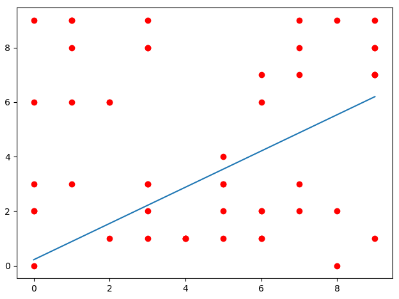# ml-python Release 2.3

The easiest way to do machine learning

Keywords
convolutional-neural-networks, gradient-descent, k-means, learn-machine-learning, library, linear-regression, logistic-regression, machine-learning, ml-python, neural-network, nonlinear-regression, python, tensorflow
MIT
Install
``` pip install ml-python==2.3 ```

# ML

This module provides for the easiest way to implement Machine Learning algorithms. It also has in-built support for graphing and optimizers based in C.

Learn the module here:

This module uses a tensorflow backend.

## Implemented Algorithms

• 2D CNN `ml.cnn`
• Basic MLP `ml.nn`
• K-Means `ml.k_means`
• Linear Regression `ml.linear_regression`
• optimized with C
• Logistic Regression `ml.logistic_regression`
• Graph Modules `ml.graph`
• Graph any function with or without data points - `from ml.graph import graph_function, graph_function_and_data`
• Nonlinear Regression `ml.regression`
• Optimizers - `ml.optimizer` optimized with C
• GradientDescentOptimizer - `from ml.optimizer import GradientDescentOptimizer`
• UNSTABLE - Character generating RNN - `ml.rnn`

### Pip installation

`pip install ml-python`

### Python installation

```git clone https://github.com/vivek3141/ml
cd ml
python setup.py install```

### Bash Installation

```git clone https://github.com/vivek3141/ml
cd ml
sudo make install```

## Examples

Examples for all implemented structures can be found in `/examples`.
In this example, linear regression is used.

First, import the required modules.

```import numpy as np
from ml.linear_regression import LinearRegression```

Then make the required object

`l = LinearRegression()`

This code below randomly generates 50 data points from 0 to 10 for us to run linear regression on.

```# Randomly generating the data and converting the list to int
x = np.array(list(map(int, 10*np.random.random(50))))
y = np.array(list(map(int, 10*np.random.random(50))))```

Lastly, train it. Set `graph=True` to visualize the dataset and the model.

`l.fit(data=x, labels=y, graph=True)`The full code can be found in `/examples/linear_regression.py`

## Makefile

A Makefile is included for easy installation.
To install using make run

`sudo make`

Note: Superuser privileges are only required if python is installed at `/usr/local/lib`